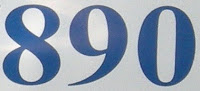## Wednesday, August 10, 2011

### 890

890 = 2 x 5 x 89.

890 has two representations as a sum of two squares: 890 = 72 + 292 = 192 + 232.

890 is a divisor of 378 - 1.

890 is 1188 in base 9.

890 is the sum of four consecutive primes: 890 = 211 + 223 + 227 + 229.WLS 890 AM is a radio station in Chicago.

Source: Wikipedia